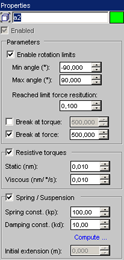Joints and axes properties.A rigid body selected for an axis is 'hard-attached' to the axis : if axis rotates/moves, corresponding rigid body will rotate/move too.Every axis in a joint has its own configuration. In order to see the configuration of a particular axis, simply select it in the active view and show the Modify panel, or click directly on the axis icon from the joint's configuration panel.

Configuring an axis:Enable rotation limits: if this option is checked, the axis can move only between the Min and Max angles. This therefore represents a mechanical constraint: Min angle: represents the minimum angle that the axis can reach. Max angle: represents the maximum angle that the axis can reach. Reached limit force restitution: indicates whether the contact between the axis and the upper or lower limits (Min or Max) is entirely absorbed (0.0) or rebounds(1.0). Break at torque: if this option is checked, the axis breaks if it is subject to torque that is higher than the given value.Break at force: if this option is checked, the axis breaks if it is subject to a force greater than the given value.Resistive torques/forces (according to axis function: rotation or translation): if option is checked, the resistant torques/forces are enabled:  Static: static resistive torque/force (axis speed = 0). Note: force/torque sensor measurements can be affected by this feature. Viscous: viscous torque/force (speed dependant). Prefer choosing small values (<=1nm/rad/s or <=1nm.m/s) for avoiding joint's instabilities. Note: force/torque sensor measurements can be affected by this feature. Note: a motor embedded on the axis can override torques/forces values. You can disable it from the motor's 'Instance Values' panel.Enable Spring/Suspension: If this option is checked, the axis has a suspension. (Option is not available for all joints): Spring constant (kp): the spring's firmness constant. Damping constant (kd): the spring's absorption constant (damping). Compute: Opens a tool window that computes Kp and Kd values according to specified information.